# 15-344/Classnotes for Thursday September 17

## Lecture Notes for September 17

DEFINITION 7 Isomorphism A graph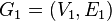$G_1 = (V_1, E_1)$ is called isomorphic to a graph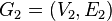$G_2 = (V_2, E_2)$ whenever there exists a bijection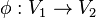$\phi:V_1 \rightarrow V_2$ such that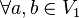$\forall a,b\in V_1$ we have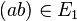$(ab)\in E_1$ if and only if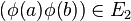$( \phi (a) \phi (b))\in E_2$.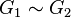$G_1\sim G_2$ means they are isomorphic to each other.

• Isomorphism does not mean two things are identical but means they are mathematically the same.

The relationship of isomorphisms:

1. Reflexive: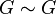$G \sim G$ A graph is isomorphic to itself

2. Symmetric: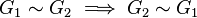$G_1 \sim G_2 \implies G_2 \sim G_1$ In other words, for every$\phi:V_1 \rightarrow V_2$ we have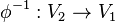$\phi ^{-1} :V_2 \rightarrow V_1$

3. Transitive: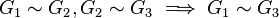$G_1 \sim G_2 , G_2 \sim G_3 \implies G_1 \sim G_3$

CLAIM If two graphs are isomorphic, then they have:

1. same number of vertices

2. same number of edges

3. vertex degrees (valencies) are the same between the two. For example, if one graph has 3 vertices of degree 2, and 2 vertices of degree 1, then the other graph should have the same

4. same number of subgraphs

5. same number of complements denoted by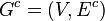$G^c = (V,E^c)$

• Complement means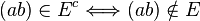$(ab)\in E^c \Longleftrightarrow (ab)\notin E$

DEFINITION 8 Subgraph A subgraph of a graph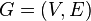$G = (V,E)$ is a graph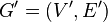$G' = (V',E')$ such that$V'\subset V$ and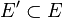$E'\subset E$.

• Checking if two graphs are isomorphic is a hard problem